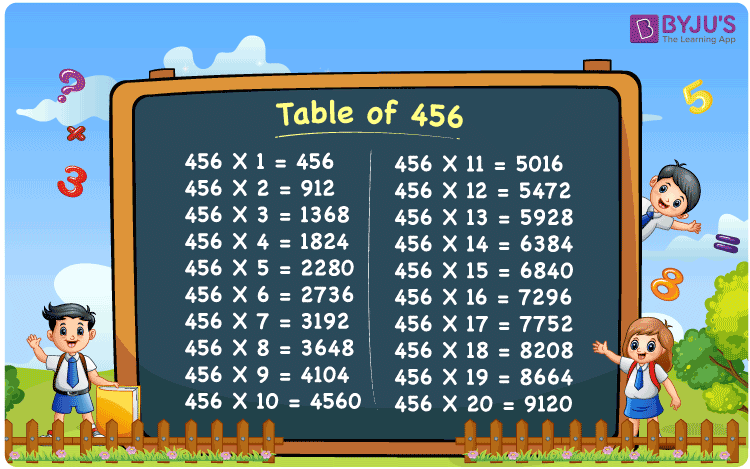Checkout JEE MAINS 2022 Question Paper Analysis : Checkout JEE MAINS 2022 Question Paper Analysis :

# Table of 456

The Multiplication Table for 456 is nothing more than the repetitive addition of the number 456. 456 is an even number that is divisible by 2 and 3 both. This implies that 456 is also divisible by 6. Observe the multiples of 456, they are divisible by 2, 3 and therefore by 6 as well. You can read more on Divisibility rules.

## Table of 456 Chart## What is the 456 Times Table?

In the table of 456, we are repeatedly adding 456 multiple times. And the table below gives a repetitive addition 10 times for the number 456.

 456 × 1 = 456 456 456 × 2 = 912 456 + 456 = 912 456 × 3 = 1368 456 + 456 + 456 = 1368 456 × 4 = 1824 456 + 456 + 456 + 456 = 1824 456 × 5 = 2280 456 + 456 + 456 + 456 + 456 = 2280 456 × 6 = 2736 456 + 456 + 456 + 456 + 456 + 456 = 2736 456 × 7 = 3192 456 + 456 + 456 + 456 + 456 + 456 + 456 = 3192 456 × 8 = 3648 456 + 456 + 456 + 456 + 456 + 456 + 456 + 456 = 3648 456 × 9 = 4104 456 + 456 + 456 + 456 + 456 + 456 + 456 + 456 + 456 = 4104 456 × 10 = 4560 456 + 456 + 456 + 456 + 456 + 456 + 456 + 456 + 456 + 456 = 4560

## Multiplication Table of 456

Given below is the multiplication table of 456 being done 20 times. The below table helps you with multiplication problems that require the tables of 456.

 456 × 1 = 456 456 × 2 = 912 456 × 3 = 1368 456 × 4 = 1824 456 × 5 = 2280 456 × 6 = 2736 456 × 7 = 3192 456 × 8 = 3648 456 × 9 = 4104 456 × 10 = 4560 456 × 11 = 5016 456 × 12 = 5472 456 × 13 = 5928 456 × 14 = 6384 456 × 15 = 6840 456 × 16 = 7296 456 × 17 = 7752 456 × 18 = 8208 456 × 19 = 8664 456 × 20 = 9120

## Solved Example on Table of 456

Example:

The best quality cashews cost Rs 456 per kg. Neena wants to buy 3 kgs of cashews. How much does she need to pay?

Solution: Rs 1368.

1 kg costs Rs 456

3 kgs costs 3 x Rs 456 = Rs 1368.

## Frequently Asked Questions on Table of 456

### Question 1: Is 456 a perfect square number?

Answer: 456 is not a perfect square number. 441 is a perfect square number.

### Question 2: Is 456 a multiple of 4?

Answer: Yes. 456 is a multiple of 4. Also the multiples of 456 are divisible by 4.